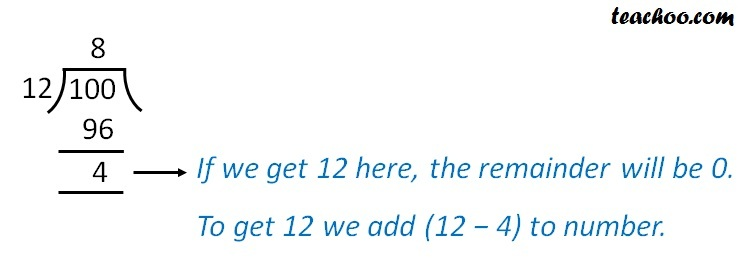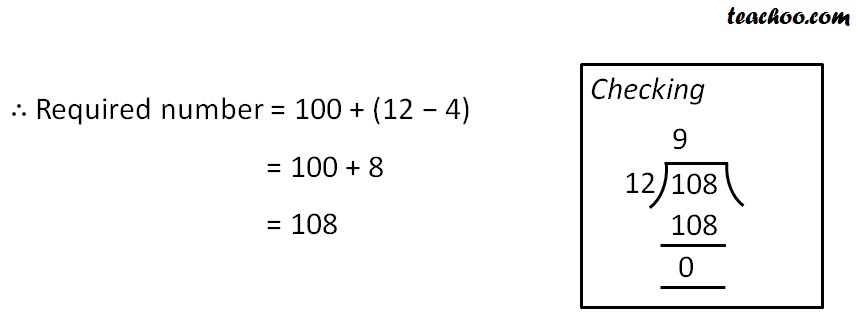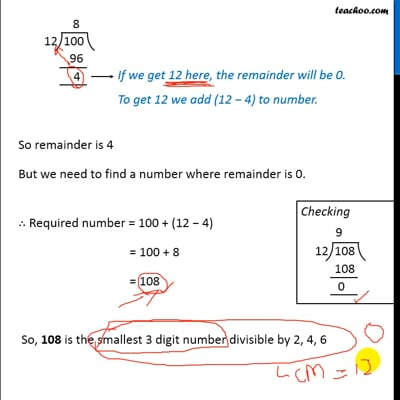Statement questions on LCM

Chapter 3 Class 6 Playing with Numbers
Concept wise

Smallest Number which divides 2, 4, 6 and leaves remainder 0

= LCM of 2, 4, 6

= 12

We need to find the smallest 3-digit number which will divide 2, 4, 6

Smallest 3 digit number = 100

We divide 100 by 12So remainder is 4

But we need to find a number where remainder is 0.So, 108 is the smallest 3 digit number divisible by 2, 4, 6This video is only available for Teachoo black users

### Transcript

What will be the smallest 3-digit number which divides 2, 4, 6 and leaves remainder 0? Smallest Number which divides 2, 4, 6 and leaves remainder 0 = LCM of 2, 4, 6 = 12 We need to find the smallest 3-digit number which will divide 2, 4, 6 Smallest 3 digit number = 100 We divide 100 by 12 If we get 12 here, the remainder will be 0. To get 12 we add (12 − 4) to number. So remainder is 4 But we need to find a number where remainder is 0. ∴ Required number = 100 + (12 − 4) = 100 + 8 = 108 So, 108 is the smallest 3 digit number divisible by 2, 4, 6